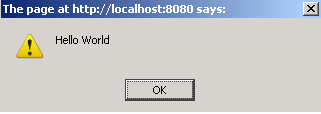Tutorials

# JSP Variable in Javascript

JSP Variable in Javascript helps you to pass jsp variable to javascript.

JSP Variable in Javascript helps you to pass jsp variable to javascript.

# JSP Variable in Javascript

JSP Variable in Javascript helps you to pass jsp variable to javascript. The JavaScript include a function that takes the defined variable from the jsp expression and display the message from variable using alert box.

Understand with Example

In this section, we are going to pass the jsp variable to javascript. You can see in the given example that we have create a function access() which accept the defined variable from the jsp expression "HelloWorld". The expression <%=str%> is used to insert the jsp variable values directly into the output. This  will display the value of string variable using the alertbox on loading the function.

Here is the code of passJspvariable.jsp



Output will be displayed as: